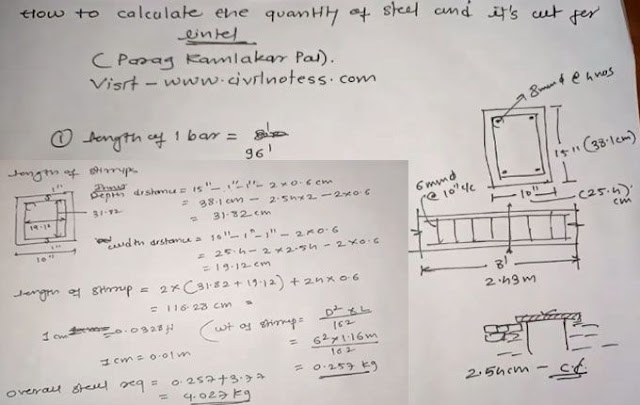How to find quantity of concrete in beam

# How to find quantity of concrete in beamThis video shows how to find quantity of concrete in a beam in 3D view. I made this video in google sketchup for civil engineering students because its very important for begineers that they should know about calculation of quantities in member like beam, column etc. calculating quantity of concrete in beam is very simple, you should just calculating the volume of beam that is given as, Volume of beam= length xwidthx height. so by using this formula we can easily calculate quantity of concrete.

Continue Reading about the "How to find quantity of concrete in beam" on the next page below